A parameter server (PS) is used to process a large number of offline and online training tasks. SMART is short for scalable multiple additive regression tree. PS-SMART is an iteration algorithm that is implemented by using a PS-based gradient boosting decision tree (GBDT). The PS-SMART Regression component supports training tasks for tens of billions of samples and hundreds of thousands of features. It can run training tasks on thousands of nodes. This component also supports multiple data formats and optimization technologies such as approximation by using histograms.

## Limits

The input data of the PS-SMART Regression component must meet the following requirements:
• Only columns of numeric data types can be used by the PS-SMART Regression component. If the type of data in the MaxCompute table is STRING, the data type must be converted first.
• If data is in the key-value format, feature IDs must be positive integers, and feature values must be real numbers. If the data type of feature IDs is STRING, you must use the serialization component to serialize the data. If feature values are categorical strings, you must perform feature engineering such as feature discretization to process the values.
• The PS-SMART Regression component can support hundreds of thousands of feature tasks. However, these tasks are resource-intensive and time-consuming. To resolve this issue, you can use GBDT algorithms to train the model. GBDT algorithms are suitable for the scenario where continuous features are used for training. You can perform one-hot encoding on categorical features to filter low-frequency features. However, we recommend that you do not perform feature discretization on continuous features of numeric data types.
• The PS-SMART algorithm may introduce randomness. For example, randomness may be introduced in the following scenarios: data and feature sampling based on data_sample_ratio and fea_sample_ratio, optimization of the PS-SMART algorithm by using histograms for approximation, and merge of a local sketch into a global sketch. The structures of trees are different when tasks run on multiple workers in distributed mode. However, the training effect of the model is theoretically the same. It is normal if you use the same data and parameters during training but obtain different results.
• If you want to accelerate training, you can set the Number of Cores parameter to a larger value. The PS-SMART algorithm starts training tasks after the required resources are provided. Therefore, the more the resources are requested, the longer you must wait.

## Considerations

When you use the PS-SMART Regression component, you must take note of the following items:
• The PS-SMART Regression component can support hundreds of thousands of feature tasks. However, these tasks are resource-intensive and time-consuming. To resolve this issue, you can use GBDT algorithms to train the model. GBDT algorithms are suitable for the scenario where continuous features are used for training. You can perform one-hot encoding on categorical features to filter low-frequency features. However, we recommend that you do not perform feature discretization on continuous features of numeric data types.
• The PS-SMART algorithm may introduce randomness. For example, randomness may be introduced in the following scenarios: data and feature sampling based on data_sample_ratio and fea_sample_ratio, optimization of the PS-SMART algorithm by using histograms for approximation, and merge of a local sketch into a global sketch. The structures of trees are different when tasks run on multiple workers in distributed mode. However, the training effect of the model is theoretically the same. It is normal if you use the same data and parameters during training but obtain different results.
• If you want to accelerate training, you can set the Number of Cores parameter to a larger value. The PS-SMART algorithm starts training tasks after the required resources are provided. Therefore, the more the resources are requested, the longer you must wait.

## Configure the component

You can configure the component by using one of the following methods:
• Use the Machine Learning Platform for AI console
Tab Parameter Description
Fields Setting Use Sparse Format Specifies whether the input data is in the sparse format. If the input data is sparse data in the key-value format, separate key-value pairs with spaces, and separate keys and values with colons (:). Example: 1:0.3 3:0.9.
Feature Columns The feature columns that are selected from the input table for training. If data in the input table is in the dense format, only the columns of the BIGINT and DOUBLE data types are supported. If data in the input table is key-value pairs in the sparse format and keys and values in the key-value pairs are of numeric data types, only columns of the STRING data type are supported.
Label Column The label column in the input table. The columns of the STRING type and numeric data types are supported. However, only data of numeric data types can be stored in the columns. For example, column values can be 0 or 1 in binary classification.
Weight Column The column that contains the weight of each row of samples. The columns of numeric data types are supported.
Parameters Setting Target Function Type The type of the objective function. Valid values: Linear Regression, Logistic Regression, Poisson Regression, Gamma Regression, and Tweedie Regression.
Tweedie Distribution Index The index for the relationship between the variance and mean value of Tweedie distribution.
Evaluation Index Type The type of the evaluation metric. Valid values: Rooted Mean Square Error, Mean Absolute Error, Negative Loglikelihood for Logistic Regression, Negative Loglikelihood for Poisson Regression, Residual Deviance for Gamma Regression, Negative Log-likelihood for Gamma Regression, Negative Log-likelihood for Tweedie Regression, and Null.
Number of Decision Trees The number of trees. The amount of training data is proportional to the number of trees.
Maximum Decision Tree Depth The default value is 5, which indicates that a maximum of 32 leaf nodes can be configured.
Data Sampling Ratio The data sampling ratio when trees are built. The sample data is used to build a weak learner to accelerate training.
Feature Sampling Ratio The feature sampling ratio when trees are built. The sample features are used to build a weak learner to accelerate training.
L1 Penalty Coefficient Controls the size of a leaf node. A larger value of this parameter indicates a more even distribution of leaf nodes. If overfitting occurs, increase the parameter value.
L2 Penalty Coefficient Controls the size of a leaf node. A larger value of this parameter indicates a more even distribution of leaf nodes. If overfitting occurs, increase the parameter value.
Learning Rate The learning rate. Valid values: (0,1).
Sketch Precision The threshold for selecting quantiles when you build a sketch. A smaller value indicates that more bins can be obtained. In most cases, the default value 0.03 is used.
Minimum Split Loss The minimum loss change required for splitting a node. A larger value indicates that node splitting is less likely to occur.
Number of Features The number of features or the maximum feature ID. If this parameter is not specified for the assessment of resource usage, the system automatically runs an SQL task to calculate the number of features or the maximum feature ID.
Global Offset The initial prediction values of all samples.
Feature Importance Type The feature importance type. Valid values: Weight, Gain, and Cover. Weight indicates the number of splits of the feature. Gain indicates the information gain provided by the feature. Cover indicates the number of samples covered by the feature on the split node.
Tuning Number of Cores The memory size of each core. By default, the system determines the value.
Memory Size per Core The memory size of each core. Unit: MB. In most cases, the system determines the memory size.
• Use commands
``````# Training
PAI -name ps_smart
-project algo_public
-DinputTableName="smart_regression_input"
-DmodelName="xlab_m_pai_ps_smart_bi_545859_v0"
-DoutputTableName="pai_temp_24515_545859_2"
-DoutputImportanceTableName="pai_temp_24515_545859_3"
-DlabelColName="label"
-DfeatureColNames="features"
-DenableSparse="true"
-Dobjective="reg:linear"
-Dmetric="rmse"
-DfeatureImportanceType="gain"
-DtreeCount="5";
-DmaxDepth="5"
-Dshrinkage="0.3"
-Dl2="1.0"
-Dl1="0"
-Dlifecycle="3"
-DsketchEps="0.03"
-DsampleRatio="1.0"
-DfeatureRatio="1.0"
-DbaseScore="0.5"
-DminSplitLoss="0"
# Prediction
PAI -name prediction
-project algo_public
-DinputTableName="smart_regression_input";
-DmodelName="xlab_m_pai_ps_smart_bi_545859_v0"
-DoutputTableName="pai_temp_24515_545860_1"
-DfeatureColNames="features"
-DappendColNames="label,features"
-DenableSparse="true"
-Dlifecycle="28"``````
Module Parameter Required Description Default value
Data parameters featureColNames Yes The feature columns that are selected from the input table for training. If data in the input table is in the dense format, only the columns of the BIGINT and DOUBLE data types are supported. If data in the input table is key-value pairs in the sparse format and keys and values in the key-value pairs are of numeric data types, only columns of the STRING data type are supported. N/A
labelColName Yes The label column in the input table. The columns of the STRING type and numeric data types are supported. However, only data of numeric data types can be stored in the columns. For example, column values can be 0 or 1 in binary classification. N/A
weightCol No The column that contains the weight of each row of samples. The columns of numeric data types are supported. N/A
enableSparse No Specifies whether data in the input table is in the sparse format. Valid values: true and false. If the input data is sparse data in the key-value format, separate key-value pairs with spaces, and separate keys and values with colons (:). Example: 1:0.3 3:0.9. false
inputTableName Yes The name of the input table. N/A
modelName Yes The name of the output model. N/A
outputImportanceTableName No The name of the table that provides feature importance. N/A
inputTablePartitions No The partitions that are selected from the input table for training. Format: ds=1/pt=1. N/A
outputTableName No The MaxCompute table that is generated. The table is a binary file. It cannot be read and can be obtained only by using the PS-SMART prediction component. N/A
lifecycle No The lifecycle of the output table. 3
Algorithm parameters objective Yes The type of the objective function. Valid values:
• reg:linear: Linear Regression
• reg:logistic: Logistic Regression
• count:poisson: Poisson Regression
• reg:gamma: Gamma Regression
• reg:tweedie: Tweedie Regression
reg:linear
metric No The evaluation metric type in the training set, which is contained in stdout of the coordinator in a logview. Valid values:
• rmse: corresponds to the Rooted Mean Square Error value of the Evaluation Index Type parameter in the console.
• mae: corresponds to the Mean Absolute Error value of the Evaluation Index Type parameter in the console.
• logistic-nloglik: corresponds to the Negative Loglikelihood for Logistic Regression value of the Evaluation Index Type parameter in the console.
• poisson-nloglik: corresponds to the Negative Loglikelihood for Poisson Regression value of the Evaluation Index Type parameter in the console.
• gamma-deviance: corresponds to the Residual Deviance for Gamma Regression value of the Evaluation Index Type parameter in the console.
• gamma-nloglik: corresponds to the Negative Log-likelihood for Gamma Regression value of the Evaluation Index Type parameter in the console.
• tweedie-nloglik: corresponds to the Negative Log-likelihood for Tweedie Regression value of the Evaluation Index Type parameter in the console.
N/A
treeCount No The number of trees. The value is proportional to the training time. 1
maxDepth No The maximum depth of a tree. Valid values: 1 to 20. 5
sampleRatio No The data sampling ratio. Valid values: (0,1]. If this parameter is set to 1.0, no data is sampled. 1.0
featureRatio No The feature sampling ratio. Valid values: (0,1]. If this parameter is set to 1.0, no features are sampled. 1.0
l1 No The L1 penalty coefficient. A larger value of this parameter indicates a more even distribution of leaf nodes. If overfitting occurs, increase the parameter value. 0
l2 No The L2 penalty coefficient. A larger value of this parameter indicates a more even distribution of leaf nodes. If overfitting occurs, increase the parameter value. 1.0
shrinkage No The learning rate. Valid values: (0,1). 0.3
sketchEps No The threshold for selecting quantiles when you build a sketch. The number of bins is O(1.0/sketchEps). A smaller value indicates that more bins can be obtained. In most cases, the default value is used. Valid values: (0,1). 0.03
minSplitLoss No The minimum loss change required for splitting a node. A larger value indicates that node splitting is less likely to occur. 0
featureNum No The number of features or the maximum feature ID. If this parameter is not specified for the assessment of resource usage, the system automatically runs an SQL task to calculate the number of features or the maximum feature ID. N/A
baseScore No The initial prediction values of all samples. 0.5
featureImportanceType No The feature importance type. Valid values:
• weight: indicates the number of splits of the feature.
• gain: indicates the information gain provided by the feature.
• cover: indicates the number of samples covered by the feature on the splitting node.
gain
tweedieVarPower No The index for the relationship between the variance and mean value of Tweedie distribution. 1.5
Tuning parameters coreNum No The number of cores used in computing. The larger the value is, the faster the computing algorithm runs. Determined by the system
memSizePerCore No The memory size of each core. Unit: MB. Determined by the system

## Example

1. Execute the following SQL statements to generate input data. In this example, input data in the key-value format is generated.
``````drop table if exists smart_regression_input;
create table smart_regression_input as
select
*
from
(
select 2.0 as label, '1:0.55 2:-0.15 3:0.82 4:-0.99 5:0.17' as features from dual
union all
select 1.0 as label, '1:-1.26 2:1.36 3:-0.13 4:-2.82 5:-0.41' as features from dual
union all
select 1.0 as label, '1:-0.77 2:0.91 3:-0.23 4:-4.46 5:0.91' as features from dual
union all
select 2.0 as label, '1:0.86 2:-0.22 3:-0.46 4:0.08 5:-0.60' as features from dual
union all
select 1.0 as label, '1:-0.76 2:0.89 3:1.02 4:-0.78 5:-0.86' as features from dual
union all
select 1.0 as label, '1:2.22 2:-0.46 3:0.49 4:0.31 5:-1.84' as features from dual
union all
select 0.0 as label, '1:-1.21 2:0.09 3:0.23 4:2.04 5:0.30' as features from dual
union all
select 1.0 as label, '1:2.17 2:-0.45 3:-1.22 4:-0.48 5:-1.41' as features from dual
union all
select 0.0 as label, '1:-0.40 2:0.63 3:0.56 4:0.74 5:-1.44' as features from dual
union all
select 1.0 as label, '1:0.17 2:0.49 3:-1.50 4:-2.20 5:-0.35' as features from dual
) tmp;``````
The generated data is shown in the following figure.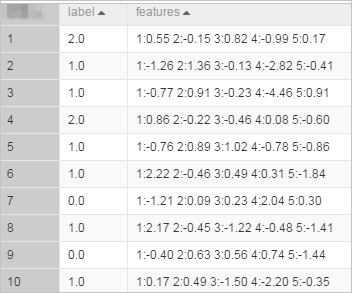2. Create the experiment shown in the following figure. For more information, see Generate a model by using an algorithm.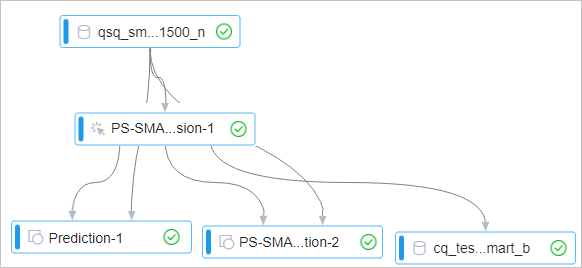3. Configure the parameters listed in the following table for the PS-SMART Regression component. Retain the default values of the parameters that are not listed in the table.
Tab Parameter Description
Fields Setting Use Sparse Format Select Use Sparse Format.
Feature Columns Select feature columns.
Label Column Select the label column.
Parameters Setting Target Function Type Set the parameter to Linear Regression.
Evaluation Index Type Set the parameter to Rooted Mean Square Error.
Number of Decision Trees Set the parameter to 5.
4. Configure the parameters listed in the following table for the unified prediction component. Retain the default values of the parameters that are not listed in the table.
Tab Parameter Description
Fields Setting Feature Columns By default, all columns in the input table are selected. Specific columns may not be used for training. These columns do not affect the prediction result.
Reserved Output Column Select the label column.
Sparse Matrix Select Sparse Matrix.
KV Delimiter Use colons (:).
KV pair delimiter Use spaces or \u0020.
5. Configure the parameters listed in the following table for the PS-SMART prediction component. Retain the default values of the parameters that are not listed in the table.
Tab Parameter Description
Fields Setting Feature Columns By default, all columns in the input table are selected. Specific columns may not be used for training. These columns do not affect the prediction result.
Reserved Output Column Select the label column.
Sparse Matrix Select Sparse Matrix.
KV Delimiter Use colons (:).
KV pair delimiter Use spaces or \u0020.
6. Run the experiment and view the prediction result of the unified prediction component.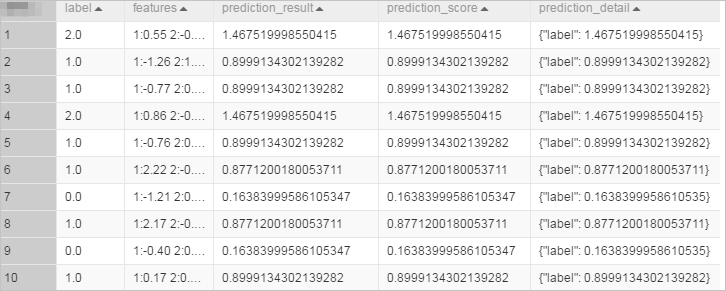7. View the prediction result of the PS-SMART prediction component.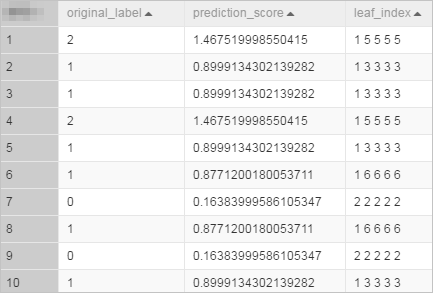The prediction_score column lists prediction values, and the leaf_index column lists the numbers of leaf nodes of the prediction.
8. Right-click the PS-SMART Regression component and choose View Data > View Output Port 3. Then, view the feature importance.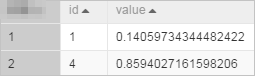The id column lists the IDs of input features. The input data in this example is key-value pairs. Therefore, the id column lists the keys in key-value pairs. The feature importance table has only two features. This indicates that only these two features are used in the split of the tree. The feature importance of other features can be considered as 0. The value column is the feature importance type. The default value of this parameter is gain, which indicates the sum of information gains provided by the feature for the model.